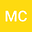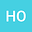loading page

Anisotropic nonlinear problem of infinite order with variables exponents and $L^1$ data
••hakima ouyahya
Université Moulay Ismail Faculté des Sciences
Author Profile## Abstract

In this paper, we prove the existence of solutions for the strongly nonlinear equation of the type $$Au+g(x,u)=f$$ where $A$ is an elliptic operator of infinite order from a functional Sobolev spaces of infinite order with variables exponents to its dual. $g(x, s)$ is a lower order term satisfying essentially a sign condition on s and the second term f belongs to $L^1(\Omega)$.